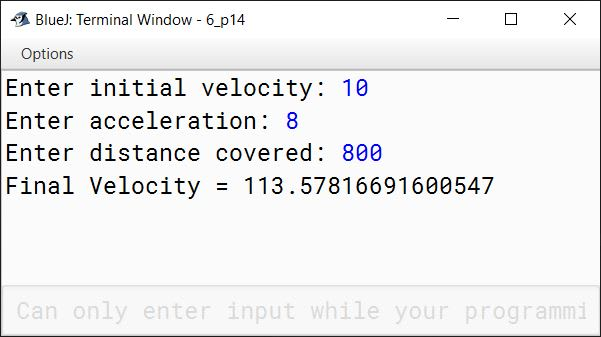# Mathematical Library Methods

## Multiple Choice Questions

#### Question 1

What will be the output of Math.cbrt(-125)?

1. 5.0
2. 0.0
3. -5.0 ✓
4. error — can't use Math.cbrt() on a negative number

#### Question 2

Which Java package includes the Math class?

1. java.io
2. java.lang ✓
3. java.util
4. java.sys

#### Question 3

Give the output of Math.sqrt(x); when x = 9.0

1. 3
2. 3.0 ✓
3. 3.00
4. All of these

#### Question 4

Give the output of Math.ceil(-0.6).

1. -1.6
2. -1.5
3. -1.0
4. -0.0 ✓

#### Question 5

Give the output of Math.ceil(-46.6).

1. -46.6
2. -46.5
3. -47.0
4. -46.0 ✓

#### Question 6

Give the output of Math.abs(x); when x = -9.99

1. -9.99
2. 9.99 ✓
3. 0.99
4. None of these

#### Question 7

Which of the following is a method to find the square root of a number?

1. FindSquareroot(x)
2. Sqrt(x)
3. Math.Square(x)
4. Math.sqrt(x) ✓

#### Question 8

What will be the output of Math.pow(3, 0)?

1. 0.0
2. 1.0 ✓
3. 3.0
4. -1.0

#### Question 9

Math.random() returns a double value r such that ...........

1. 0.0 <= r < 1.0 ✓
2. 0.0 <= r <= 1.0
3. 0.0 < r <= 1.0
4. 0.0 < r < 1.0

#### Question 10

What will be the output of Math.floor(-20.10)?

1. -20.0
2. -21.0 ✓
3. 20
4. 21

#### Question 11

What will be the output of Math.round(0.5)?

1. 0.0
2. 0
3. 1 ✓
4. 1.0

#### Question 12

What will be the output of Math.abs(-0)?

1. 0.0
2. 0 ✓
3. -0
4. +0

#### Question 13

Given the following statements:

int min = 1, max = 10;
int range = max - min + 1;
int num = (int) (range * Math.random() + min);

The value of num will be in integer such that ...........

1. 1 <= num <= 10 ✓
2. 1 <= num < 10
3. 1 < num <= 10
4. 1 < num < 10

## Assignment Questions

#### Question 1

How do user-defined methods differ from library methods?

A user-defined method is a method defined by the user whereas library methods or built-in methods are the methods created by the developers of Java which are available in the form of packages.

#### Question 2

Distinguish between Math.ceil() and Math.floor() methods.

Math.ceil( )Math.floor( )
Returns the smallest double value that is greater than or equal to the argument and is equal to a mathematical integerReturns the largest double value that is less than or equal to the argument and is equal to a mathematical integer.
double a = Math.ceil(65.5);
In this example, a will be assigned the value of 66.0 as it is the smallest integer greater than 65.5.
double b = Math.floor(65.5);
In this example, b will be assigned the value of 65.0 as it is the largest integer smaller than 65.5.

#### Question 3

What is wrong with the following statements? Explain.

i. result = (5/10) * Math.sqrt( a );

(5/10) will lead to integer division as both numerator and denominator are integers. So result of (5/10) will be 0 instead of 0.5 and the entire expression will always result in 0.

ii. result = math.sqrt(b * b - 4 * a * c) / ( 2 * a );

math should be written as Math. As Java is case-sensitive so it treats math and Math differently.

#### Question 4

Explain the following Math functions in Java:

i. Math.abs()

Returns the absolute value of its argument. Its return type is same as the type of its arguments. For example, Math.abs(-5) will return 5.

ii. Math.sqrt()

Returns the square root of its argument as a double value. For example, Math.sqrt(25) will return 5.0.

iii. Math.cbrt()

Returns the cube root of its argument as a double value. For example, Math.cbrt(27) will return 3.0.

iv. Math.random()

Returns a positive double value, greater than or equal to 0.0 and less than 1.0.

v. Math.round()

Rounds off its argument to the nearest mathematical integer and returns its value as an int or long type. If argument is float, return type is int, if argument is double, return type is long. At mid-point, it returns the higher integer. For example, Math.round(2.5) will return 3.

vi. Math.ceil()

Returns the smallest double value that is greater than or equal to the argument and is equal to a mathematical integer. For example, Math.ceil(65.5) will return 66.0.

#### Question 5

Write Java expressions for the following:

i. The square root of a + 5b3

Math.sqrt(a + 5 * Math.pow(b, 3))

ii. The cube root of x3 + y3 + z3

Math.cbrt(x*x*x + y*y*y + z*z*z);

iii. The square root of b2 + 4ac

Math.sqrt(b * b + 4 * a * c)

#### Question 6

Write the following as Java expressions:

i. $\sqrt{x^2 + 5y^3}$

Math.cbrt(x * x + 5 * y * y * y)

ii. $\lvert x + y\rvert$

Math.abs(x + y)

iii. $\lvert x^3 + y^2 -2xy\rvert$

Math.abs(Math.pow(x, 3) + Math.pow(y, 2) - 2*x*y)

iv. $\dfrac{\pi}{6}(z^4 - 2\pi)$

Math.PI / 6*(Math.pow(z, 4) - 2*Math.PI)

v. $\sqrt{z^2 - \pi}$

Math.cbrt(z*z - Math.PI)

vi. $\sqrt{x^3 - y^3}$

Math.pow(x*x*x - y*y*y, 1/4)

vii. $\cfrac{amount*rate}{1 - \cfrac{1}{(1 + rate)^n}}$

(amount*rate) / (1 - (1 / Math.pow(1+rate, n)))

viii. $\dfrac{(-b + \sqrt{b^2 - 4ac})}{2a}$

(-b + Math.sqrt(b*b - 4*a*c)) / (2*a)

ix. $\sqrt{\dfrac{1}{LC} - \dfrac{R^2}{4C^2}}$

Math.pow((1 / L*C) - ((R*R)/(4*C*C)), 1/4)

#### Question 7

Write valid statements for the following in Java:

i. Print the rounded off value of 14.49

System.out.println(Math.round(14.49));

ii. Print the absolute value of -0.09

System.out.println(Math.abs(-0.09));

iii. Print the largest of -67 and -50

System.out.println(Math.max(-67, -50));

iv. Print the smallest of -56 and -57.4

System.out.println(Math.min(-56, -57.4));

v. Print a random integer between 25 and 35

int range = 35 - 25 + 1;
int num = (int)(range * Math.random() + 25);
System.out.println(num);

vi. Print 47.5 raised to the power 6.3

System.out.println(Math.pow(47.5, 6.3));

vii. Print minimum of -4, -7

System.out.println(Math.min(-4, -7));

#### Question 8

Write a program in Java to find the maximum of three numbers using Math.max() method.

import java.util.Scanner;

public class KboatGreatestNumber
{
public static void main(String args[]) {

Scanner in = new Scanner(System.in);

System.out.print("Enter First Number: ");
int a = in.nextInt();
System.out.print("Enter Second Number: ");
int b = in.nextInt();
System.out.print("Enter Third Number: ");
int c = in.nextInt();

int g = Math.max(a, b);
g = Math.max(g, c);

System.out.println("Greatest Number = " + g);
}
}
##### Output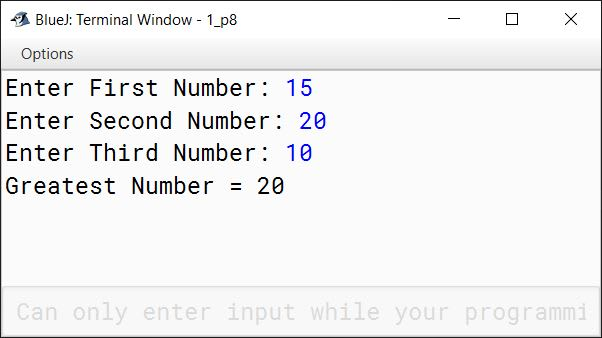#### Question 9

Write a program to print:

i. x to the power y

ii. the square root of y

The values of x and y are 81 and 3, respectively.

public class KboatNumber
{
public static void main(String args[]) {
int x = 81;
int y = 3;

double r1 = Math.pow(x, y);
System.out.println("x to the power of y = " + r1);

double r2 = Math.sqrt(y);
System.out.println("Square root of y = " + r2);
}
}
##### Output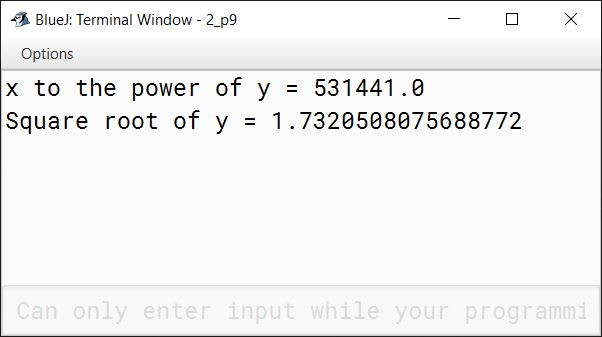#### Question 10

Write a program to compute and display the value of expression:

$\dfrac{1}{x^2} + \dfrac{1}{y^3} + \dfrac{1}{z^4}$

where, the values of x, y and z are entered by the user.

import java.util.Scanner;

public class KboatExpression
{
public static void main(String args[]) {

Scanner in = new Scanner(System.in);
System.out.print("Enter x: ");
int x = in.nextInt();
System.out.print("Enter y: ");
int y = in.nextInt();
System.out.print("Enter z: ");
int z = in.nextInt();

double result = 1 / Math.pow(x, 2) + 1 / Math.pow(y, 3) + 1 / Math.pow(z, 4);

System.out.println("Result = " + result);
}
}
##### Output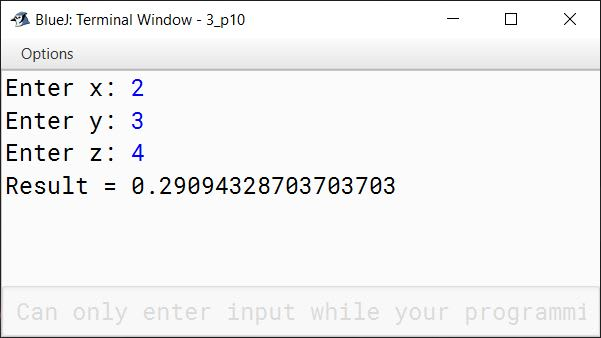#### Question 11

Write a program to generate random integers in the following ranges:

i. 10 to 20 (both inclusive)
ii. 25 to 50 (both inclusive)

public class KboatRandom
{
public static void main(String args[]) {
int min = 10, max = 20;
int range = max - min + 1;
int num = (int)(range * Math.random() + min);
System.out.println("Random number in the range 10 to 20: " + num);

min = 25;
max = 50;
range = max - min + 1;
num = (int)(range * Math.random() + min);
System.out.println("Random number in the range 25 to 50: " + num);
}
}
##### Output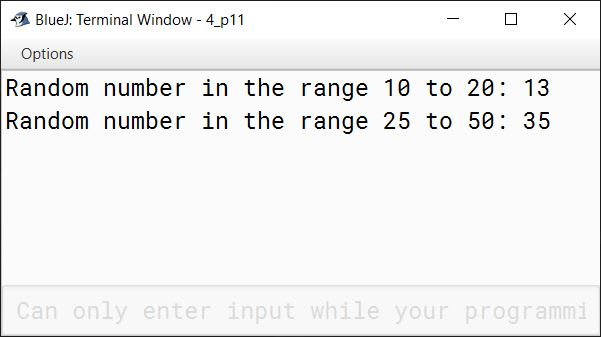#### Question 12

Write a program that accepts a number x and then prints:

x0, x1, x2, x3, x4, x5

import java.util.Scanner;

public class KboatXPower
{
public static void main(String args[]) {
Scanner in = new Scanner(System.in);
System.out.print("Enter x: ");
int x = in.nextInt();
System.out.print(Math.pow(x, 0) + ", ");
System.out.print(Math.pow(x, 1) + ", ");
System.out.print(Math.pow(x, 2) + ", ");
System.out.print(Math.pow(x, 3) + ", ");
System.out.print(Math.pow(x, 4) + ", ");
System.out.print(Math.pow(x, 5));
}
}
##### Output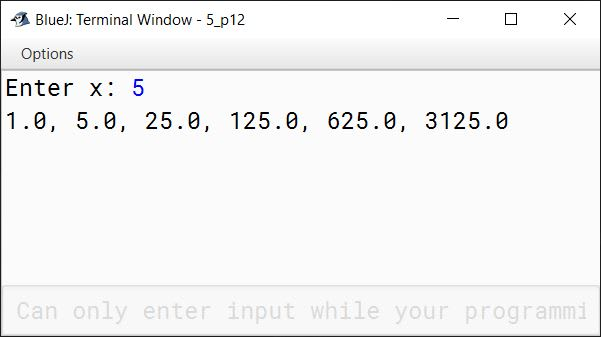#### Question 13

Write the equivalent Java statements for the following, by using the mathematical functions:

i. Print the positive value of -999.

System.out.println(Math.abs(-999));

ii. Store the value -3375 in a variable and print its cube root.

int a = -3375;
System.out.println(Math.cbrt(a));

iii. Store the value 999.99 in a variable and convert it into its closest integer that is greater than or equal to 999.99.

double a = 999.99;
System.out.println(Math.round(a));

#### Question 14

Write a program in Java to compute the final velocity of a vehicle using the following formula:

$v^2 = u^2 + 2as$

where, u = initial velocity, a = acceleration and s = distance covered; they are entered by the user.

import java.util.Scanner;

public class KboatVelocity
{
public static void main(String args[]) {
Scanner in = new Scanner(System.in);
System.out.print("Enter initial velocity: ");
double u = in.nextDouble();
System.out.print("Enter acceleration: ");
double a = in.nextDouble();
System.out.print("Enter distance covered: ");
double s = in.nextDouble();
double v = Math.sqrt(u * u + 2 * a * s);
System.out.println("Final Velocity = " + v);
}
}
##### Output